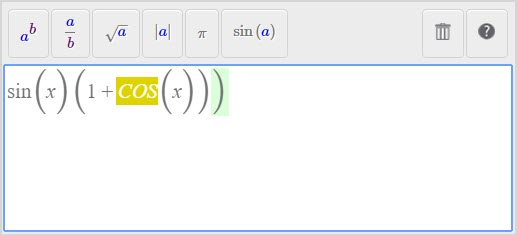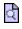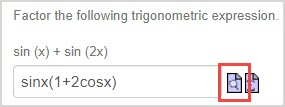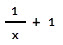# Enter my response with proper math syntax

Proper mathematical syntax is required while entering your response to questions whether you're using Text Mode or Symbol Mode for your responses.

TIP: Check out Choose a math entry mode to learn about the differences between these two math entry modes.

NOTE: When using Symbol Mode, the Equation Editor employs a Syntax Checker to support you in entering proper math syntax.Check out Work with the Syntax Checker to learn how to make the most of this tool.

The following types of mathematical entries require particular syntax styling in order for Möbius to correctly interpret your response:

• Mathematical expressions
• Variable names
• Function and operator syntax

Some notation applies to all of Möbius, while others apply specifically to questions that require you to respond with Maple Syntax.

Maple Syntax — The language that the MapleTM math engine uses to interpret mathematical expressions.

IMPORTANT: Some questions in Möbius require you to enter Maple Syntax for your mathematical expressions. If it isn't specified by your instructor whether you should be using Maple Syntax or not, preview your entered response whenever possible by clicking the preview iconbeside your Text Mode answer region.Using the preview ensures that your entry is being properly interpreted by Möbius. If your preview is blank or doesn't appear as expected, it may be because you aren't using the correct syntax.## Mathematical expression syntax

When entering formulas and expressions as your response, use standard mathematical notation that's similar to that used by a graphing calculator (Example(x^2-2*x+1)*2*sin(x)*(x^2+1)*e^(-x^2) is accepted).

Here are some notes on using mathematical expression syntax when entering your response:

• Place the argument of a function in parentheses () (Example — enter sqrt(3x) not sqrt 3x, which is interpreted as (sqrt(3))*x)
• Be sure to include parentheses () (Example — the expression 1/(x+1) is different from 1/x+1 which Möbius interprets as)

## Variable name syntax

The variable(s) in your response must be identical—this includes case sensitivity—to the variable(s) used in the question statement.

If these don't match, your response will be graded as incorrect (Example — if the question uses the variable t, but you've entered a response that uses T, your response won't match the defined correct answer and will be graded as incorrect).

## Function and operator syntax

Functions and operators require specific syntax when they're used within your response.

The required syntax varies depending on whether you're required to respond using Möbius syntax or Maple syntax.

NOTE: In general, you can assume that you should be using Möbius syntax unless your instructor has provided specific instructions that you're required to respond using Maple syntax.

TIP: Remember to preview your response whenever possible using the preview iconto ensure that the syntax that you're using is properly interpreted by Möbius.

### Arithmetic

Arithmetic Syntax for Möbius or Maple
Subtraction -
Multiplication *
Division /
Exponentiation ^

TIP: Alternatively, for multiplication with Möbius syntax, you can use:

• Two letters with a space (Examplex y)
• A letter and a number with or without a space (Example2x or 2 x)

However, for multiplication with Maple syntax, you must include an asterisk (*) (Example2*x and not 2x; x*y and not xy; x*y and not x y).

### Trigonometric functions

Function Syntax for Möbius or Maple
Sine sin
Cosine cos
Tangent tan
Inverse sine arcsin
Inverse cosine arccos
Inverse tangent arctan
Secant sec
Cosecant csc
Cotangent cot

NOTE: Arguments of trigonometric functions are evaluated in radians. The results of the inverse trigonometric functions are evaluated in radians.

### Hyperbolic trigonometric functions

Function Möbius syntax Maple syntax
Hyperbolic sine hypsin sinh
Hyperbolic cosine hypcos cosh
Hyperbolic tangent hyptan tanh
Hyperbolic secant hypsec sech
Hyperbolic cosecant hypcsc csch
Hyperbolic cotangent hypcot coth
Inverse hyperbolic sine archypsin arcsinh
Inverse hyperbolic secant archypsec arcsech
Inverse hyperbolic cosecant archypcsc archcsch
Inverse hyperbolic cotangent archypcot arccoth

NOTE: Arguments of trigonometric functions are evaluated in radians. The results of the inverse trigonometric functions are evaluated in radians.

### Numbers and constants

N/A* — Not available
Number or constant Möbius syntax Maple syntax
2.71828... e exp(1)
3.14159... Pi Pi
Scientific notation E EN/A* I

TIP: The E for scientific notation is entered between the coefficient and the order of magnitude (Example290,000,000 would be 2.9E8).

NOTE: For the square root of -1, it's a capital letter i.

### Functions

N/A* — Not available
Function Möbius syntax Maple syntax
Limit of a function ƒ N/A* limit(f, x=a)
Derivative or partial derivative of a function ƒ N/A* diff(f, x)
Integral of a function ƒ N/A* int(f, x)
Absolute value abs(x) abs(x)
Square root sqrt(x) or x^(1/2) sqrt(x) or x^(1/2)
Exponential e^x exp(x)
Logarithm base b N/A* log[b](x)
Logarithm base 10 log(x) log10(x)
Natural logarithm (base e) ln(x) log(x) or ln(x)

IMPORTANT: When you're required to respond using Maple syntax, your instructor should indicate in their question statement that:

• log in Maple is interpreted as log base 10